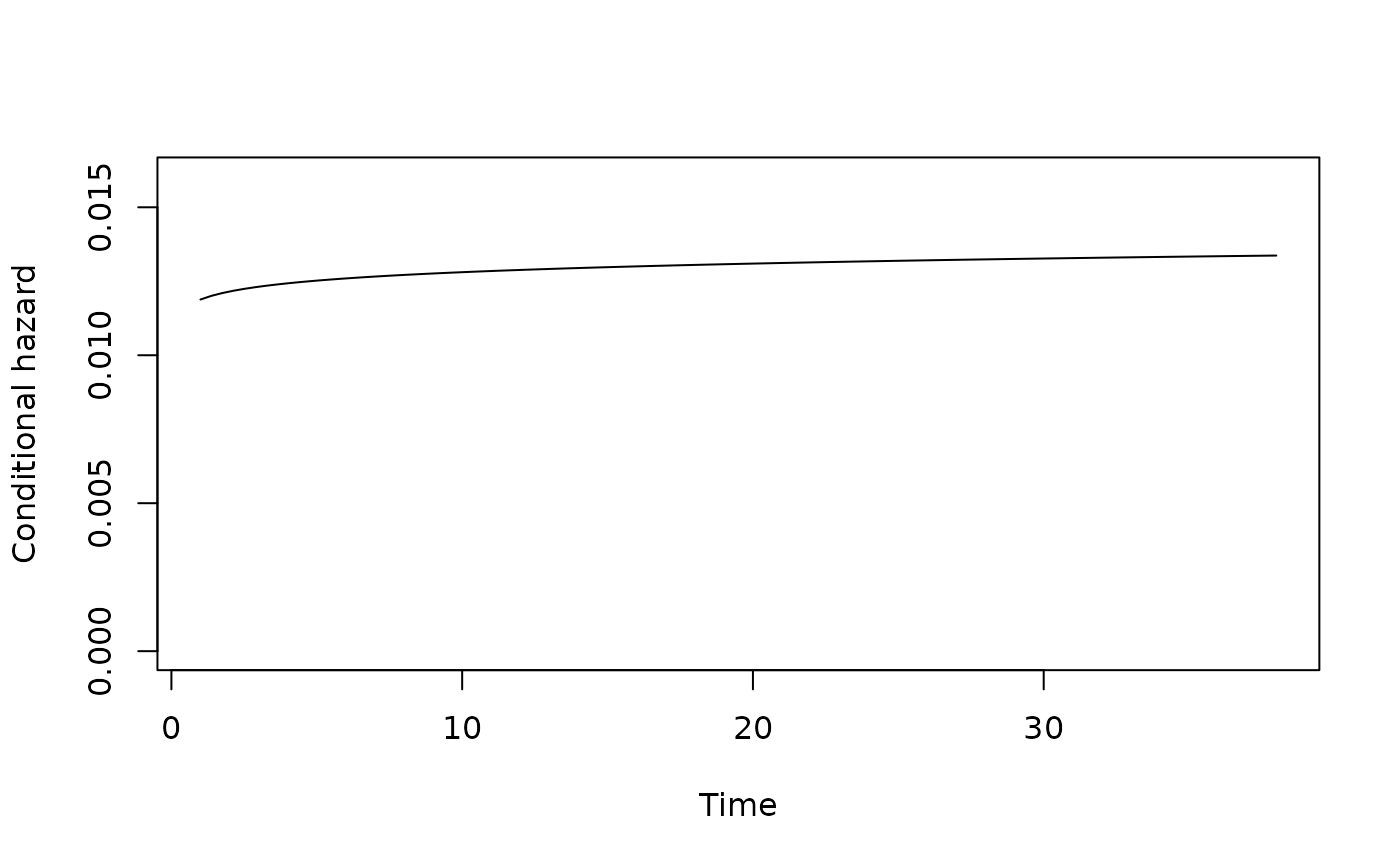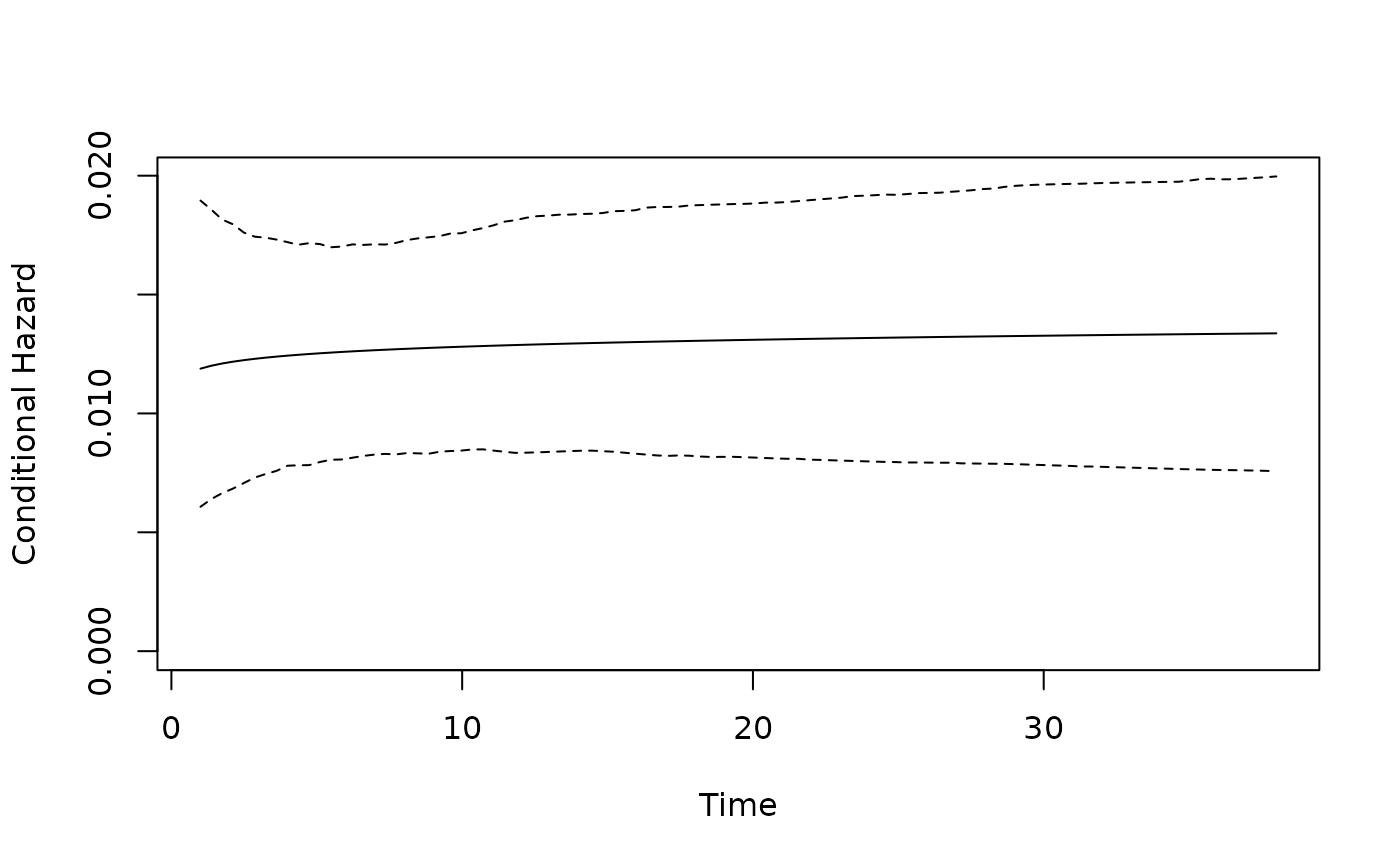plot_hazard plots the shape of estimated hazard function in respect to duration, given a set of values for the duration and risk equations covariates. Confidence intervals are provided through simulation.

plot_hazard(x, t = NULL, ci = TRUE, n = 1000, xvals = NULL,
zvals = NULL, ...)

## Arguments

x An object of class spdur Time values at which to evaluate hazard function, e.g. c(1:50). Defaults to 1 through 1.2 * maximum duration value in data. Compute simulation-based confidence interval? Number of simulations to use for CI, defaults to 1,000. A vector of values for the duration equation variables, in the same order as the duration equation in x. Defaults to means. A vector of values for the risk equation variables, in the same order as the risk equation in x. Defaults to means. Additional parameters passed to plot.

sepplot
# Get model estimates
plot_hazard(model.coups, ci = FALSE)plot_hazard(model.coups, ci = TRUE)